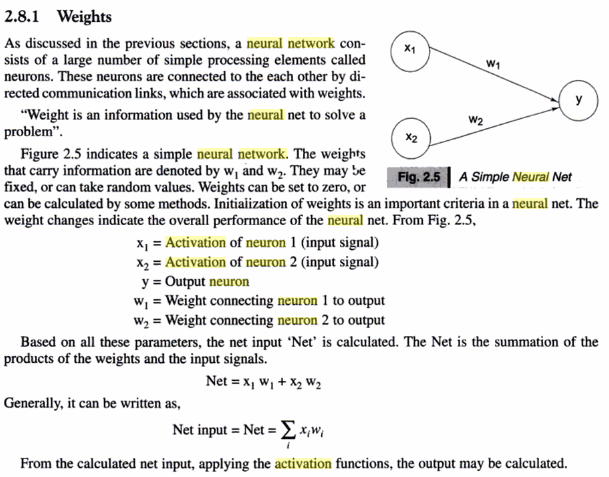# In neural network literature, which one is activation?

Consider an MLP with one hidden layer. Mathematically, denoting the input as $x$, the output, say, $y$ is calculated by $$y = \sigma(W_2a_2 + b_2)$$ where $$a_2 = \sigma(z_2), \qquad z_2 = W_1x+b_1.$$ What confuses me is which one is called activation, for example, in the second layer? Is the activated signal $a_2$ called the activation of 2-nd layer? Or is $z_2$, the signal before activated, called so?

A 'layer' does not have an activation. Each individual neuron has an activation.

The state of a neuron is it's bias + all incoming connections (weight * activation from source neuron). So that's $z_2$.

The activation is the state of a neuron passed through an activation function. So that's $a_2$. As $\sigma()$ is the activation function.

Related: 

The answer below seems contradictory, however (logically) it makes sense to call the activation of a neuron the value that is received from the activation function. References to support my claim:As you see, the value neuron y is getting from x1 is called the 'activation of neuron1', meaning its output - thus the value received after the activation function. [Source]

• Note that sometimes the activations of a group of neurons are called also the activation vector. So, the output of a layer can also be called activation, but this activation is a vector. – Hossein Apr 6 '17 at 10:27

I've seen people calling both activation, but the input of the activation function ($z_2$) seems more formal.

For example in Pattern Recognition and Machine Learning (the following $a_j$ and $z_j$ are not the same as in the question)this batch normalization paper from Google (where the word "activation" is mentioned 42 times to denote the input of the activation function), and also this layer normalization paper by Hinton et al.

• Great mentioning the Batch Normalization paper! – Spectral Aug 5 '18 at 22:06

Normally, the output of each neuron after performing the activation function is called the activation of that neuron. So, in your example, $a_2$ is the activation of the hidden neuron and $y$ is the activation of the output neuron.